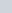A spherical ball of radius 3 cm is melted and recast into three spherical balls.
Question:

A spherical ball of radius 3 cm is melted and recast into three spherical balls. The radii of the two of the balls are 1.5 cm and 2 cm respectively. Determine the diameter of the third ball.

Solution:

We have one spherical ball of radius 3 cm

So, its volume $=\frac{4}{3} \pi(3)^{3}$……(a)

It is melted and made into 3 balls.

The first ball has radius 1.5 cm

So, its volume $=\frac{4}{3} \pi(1.5)^{3}$……(b)

The second ball has radius 2 cm

So, its volume $=\frac{4}{3} \pi(2)^{3}$……..(c)

We have to find the radius of the third ball.

Let the radius of the third ball beThe volume of this third ball $=\frac{4}{3} \pi(r)^{3}$…….(d)

We know that the sum of the volumes of the 3 balls formed should be equal to the volume of the given spherical ball.

Using equations (a), (b), (c) and (d)

$\frac{4}{3} \pi(r)^{3}+\frac{4}{3} \pi(1.5)^{3}+\frac{4}{3} \pi(2)^{3}=\frac{4}{3} \pi(3)^{3}$

$\Rightarrow(r)^{3}+(1.5)^{3}+(2)^{3}=(3)^{3}$

$r^{3}=27-8-\frac{27}{8}$

$r^{3}=\frac{7 \times 27-64}{8}$

$r^{3}=\frac{125}{8}$

$\Rightarrow r=\frac{5}{2}=2.5 \mathrm{~cm}$

Hence the diameter of the third ball should be 5 cm